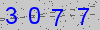# Reporting correlations

Correlation analysis—most commonly Pearson correlation analysis—is a valuable statistical technique for establishing 1) whether there is a relationship between variables and the 2) strength of that relationship.

However, there is often confusion on the meaning of the statistical results for such an analysis and the conventional reporting procedures. We aim to correct this confusion.

First, the best possible step is to reference the journal guidelines. Journals where correlation analysis is popular will often specifically state the required reporting measures or reference reporting measures from another organization. In this case, follow these explicit guidelines.

Second, you can adopt the following practice in the absence of any explicit instructions from the journal. These are the elements that must be reported:

• Correlation coefficient (r) or the coefficient of determination (r2)
• p values are required in hypothesis testing to determine the existence of a statistically significant relationship. Be careful not to imply that the p-value has any sort of reflection on the strength or relevance of the relationship.
• Degrees of freedom
• Many journals now require this as the degrees of freedom provides necessary information regarding the amount of data that was used to produce the estimate of the statistical parameter.
• Sample size
• The sample size does not necessarily need to be reported alongside the other statistics, but the sample size used for the correlation must be clear from the experimental design.

Finally, it is wise to include a scatter plot for any correlation analysis. Given that the strength of the relationship is a subjective valuation, the reader should be given an opportunity to gauge the data used to calculate the correlation coefficient. The author should not hide certain data anomalies that might contribute to a statistically significant correlation, such as clustering of data points at the extremes of the relationship. It is also important to include the regression equation generated during the correlation analysis on this plot.

Security Code*